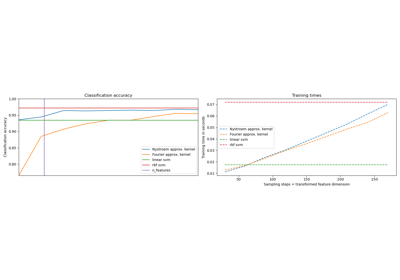# sklearn.kernel_approximation.RBFSampler¶

class sklearn.kernel_approximation.RBFSampler(*, gamma=1.0, n_components=100, random_state=None)[source]

Approximates feature map of an RBF kernel by Monte Carlo approximation of its Fourier transform.

It implements a variant of Random Kitchen Sinks.

Read more in the User Guide.

Parameters
gammafloat

Parameter of RBF kernel: exp(-gamma * x^2)

n_componentsint

Number of Monte Carlo samples per original feature. Equals the dimensionality of the computed feature space.

random_stateint, RandomState instance or None, optional (default=None)

Pseudo-random number generator to control the generation of the random weights and random offset when fitting the training data. Pass an int for reproducible output across multiple function calls. See Glossary.

Attributes
random_offset_ndarray of shape (n_components,), dtype=float64

Random offset used to compute the projection in the n_components dimensions of the feature space.

random_weights_ndarray of shape (n_features, n_components), dtype=float64

Random projection directions drawn from the Fourier transform of the RBF kernel.

Notes

See “Random Features for Large-Scale Kernel Machines” by A. Rahimi and Benjamin Recht.

 “Weighted Sums of Random Kitchen Sinks: Replacing minimization with randomization in learning” by A. Rahimi and Benjamin Recht. (https://people.eecs.berkeley.edu/~brecht/papers/08.rah.rec.nips.pdf)

Examples

>>> from sklearn.kernel_approximation import RBFSampler
>>> from sklearn.linear_model import SGDClassifier
>>> X = [[0, 0], [1, 1], [1, 0], [0, 1]]
>>> y = [0, 0, 1, 1]
>>> rbf_feature = RBFSampler(gamma=1, random_state=1)
>>> X_features = rbf_feature.fit_transform(X)
>>> clf = SGDClassifier(max_iter=5, tol=1e-3)
>>> clf.fit(X_features, y)
SGDClassifier(max_iter=5)
>>> clf.score(X_features, y)
1.0


Methods

 fit(X[, y]) Fit the model with X. fit_transform(X[, y]) Fit to data, then transform it. get_params([deep]) Get parameters for this estimator. set_params(**params) Set the parameters of this estimator. Apply the approximate feature map to X.
__init__(*, gamma=1.0, n_components=100, random_state=None)[source]

Initialize self. See help(type(self)) for accurate signature.

fit(X, y=None)[source]

Fit the model with X.

Samples random projection according to n_features.

Parameters
X{array-like, sparse matrix}, shape (n_samples, n_features)

Training data, where n_samples in the number of samples and n_features is the number of features.

Returns
selfobject

Returns the transformer.

fit_transform(X, y=None, **fit_params)[source]

Fit to data, then transform it.

Fits transformer to X and y with optional parameters fit_params and returns a transformed version of X.

Parameters
X{array-like, sparse matrix, dataframe} of shape (n_samples, n_features)
yndarray of shape (n_samples,), default=None

Target values.

**fit_paramsdict

Returns
X_newndarray array of shape (n_samples, n_features_new)

Transformed array.

get_params(deep=True)[source]

Get parameters for this estimator.

Parameters
deepbool, default=True

If True, will return the parameters for this estimator and contained subobjects that are estimators.

Returns
paramsmapping of string to any

Parameter names mapped to their values.

set_params(**params)[source]

Set the parameters of this estimator.

The method works on simple estimators as well as on nested objects (such as pipelines). The latter have parameters of the form <component>__<parameter> so that it’s possible to update each component of a nested object.

Parameters
**paramsdict

Estimator parameters.

Returns
selfobject

Estimator instance.

transform(X)[source]

Apply the approximate feature map to X.

Parameters
X{array-like, sparse matrix}, shape (n_samples, n_features)

New data, where n_samples in the number of samples and n_features is the number of features.

Returns
X_newarray-like, shape (n_samples, n_components)

## Examples using sklearn.kernel_approximation.RBFSampler¶